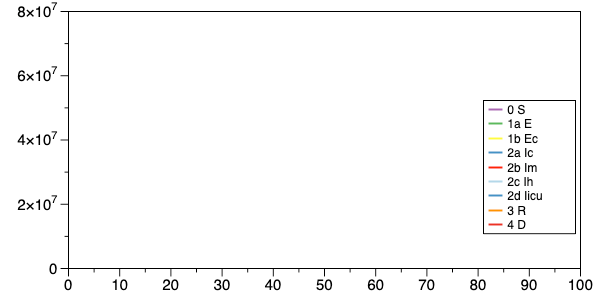3

# BayesiaLab Tech Talk: Epidemic Modeling with Bayesian Networks

Compartmental models represent the most common approach for characterizing the development of an epidemic. In an earlier webinar, we introduced a compartmental S-I-R-D model and created a highly-simplified Bayesian network to illustrate the principles. Given its great relevance, we believe the topic warrants a more detailed explanation beyond the initial "toy model."

For the purpose of this BayesiaLab Tech Talk, we present a more comprehensive S-E-I-R-D model. Each letter denotes a compartment (or state) of individuals in a population:

• S: number of susceptible
• E: number of exposed
• I: number of infected
• R: number recovered

Additionally, we further differentiate within the states of exposed and infected to account for contagiousness and disease severity.In standard models, a set of differential equations describes how individuals move between the compartments/states. In this Tech Talk, we implement the differential equations as probabilistic, temporal relationships between nodes in a Bayesian network.

While we often use fictional values in webinars to emphasize methodology over the subject matter, we take a different approach here: The numerical values and parameters presented in this Tech Talk are derived from current COVID-19 observations in France. As a result, the model attempts to represent the actual pandemic situation in France and forecast the pandemic progression.

3replies Oldest first
• Oldest first